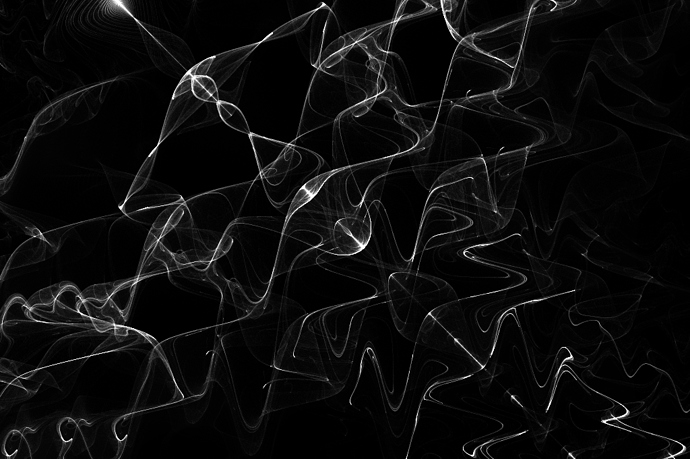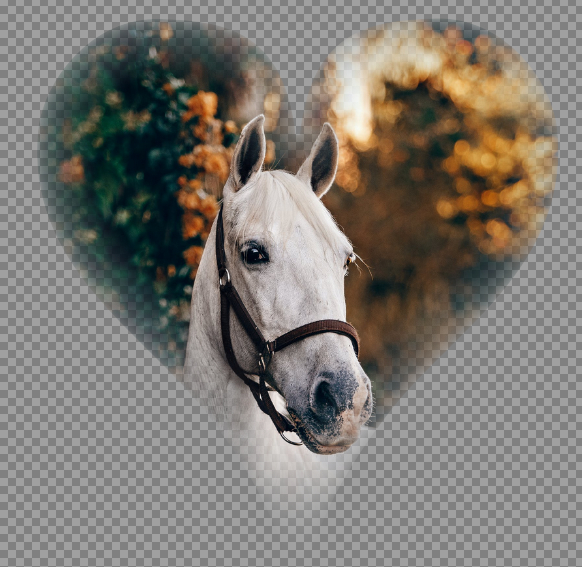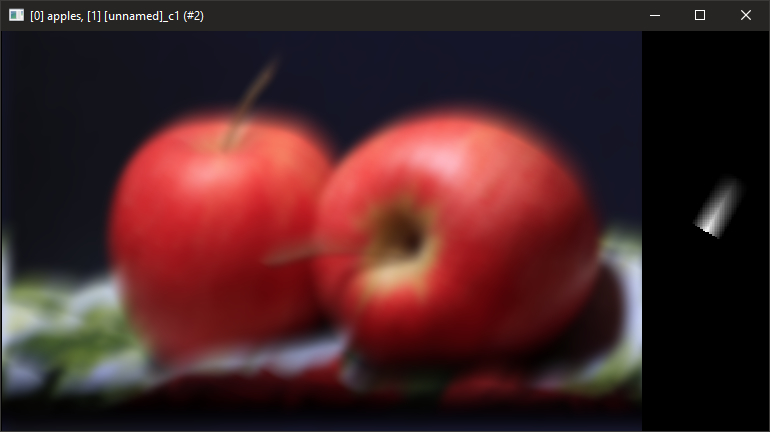# Reptorian's G'MIC Code Workshop

## Recommended Posts

3 hours ago, Seerose said:

❤️ Dear

Gmic collection. zip, I opened it, I don't know where the folder is.

That .zip file is a collection of note in gmic code form. It's only for scripters and programmers. Some of them can be copied and pasted into Custom Code gui filter.

If you really want to code within g'mic scripting, I would start with downloading KDE Kate and open those files with KDE Kate (That is what I use), look into Custom Code filters under Various under gmic-qt plugin under paint.net. Then, use the g'mic reference, and read tutorials on how to code. In a way, it's a lot easier to code in filters with gmic-qt than it is to work with c# or c++.  Another source you can use to learn it is to read G'MIC-QT STDLIB or look at the g'mic-community repository. Almost every codes I done for g'mic-qt is found within reptorian.gmic. If you need more help, I would ask discuss.pixls.us forum, and they'll help you understand how everything works better than I can.

Edited by Reptillian
•1

G'MIC Filter Developer

##### Share on other sites

❤️ Dear

Thank you very much for detailed information.

Unfortunately, I'm a long way from being able to do that.

Thanks again.Live as if you were to die tomorrow. Learn as if you were to live forever.

Gandhi##### Share on other sites

• 3 weeks later...

I got news. Where I'm at, hospitals are getting filled with COVID-19 patients and in fact, less than 10 counties have hospitals at 10- percent capacity. Actually, I do not think it would be wise to try to find a job when I already have a roof over me. So, I will code up a few things.

•3

G'MIC Filter Developer

##### Share on other sites

On 7/8/2020 at 7:51 AM, Reptillian said:

hospitals are getting filled with COVID-19 patients

Stay safe, stay at home if you can.

On 7/8/2020 at 7:51 AM, Reptillian said:

So, I will code up a few things.

That is good news, you are our only G'MIC coder on the forum. (I did not forget

##### Share on other sites

Here's what I have coded in. Paste it into code filter under G'MIC-QT->Various section and see what you get. You get this image:```rep_pfrac 100,1,.25,10,1,0,0,0,1,0,0,1
rep_pfrac: rep_popcorn_fractal \$*
#\$1==points_per_pixels#
#\$2==density#
#\$3==H#
#\$4==K#
#\$5==zoom#
#\$6==rotation_angle#
#\$7==origin_x#
#\$8==origin_y#
#\$9==formulamode#
rep_popcorn_fractal:
skip \${1=50},\${2=1},\${3=.05},\${4=3},\${5=1},\${6=0},\${7=0},\${8=0},\${9=0},\${10=},\${11=},\${12=},\${13=},\${14=},\${15=}
f 0 r 100%,100%,1,1
repeat \$! l[\$>]
eval \${-math_lib}"
const pts=\$1;
const density=1/\$2;
const H=\$3;
const K=\$4;
const zoom=1/\$5;
const ang=(\$6/180)*pi;
const origin_x=\$7*-1*zoom;
const origin_y=\$8*zoom;
const sd=max(w,h)/min(w,h);
const sx=w>h?sd:1;
const sy=w>h?1:sd;
const cx=w/2;
const cy=h/2;
const ocx=origin_x*cx;
const ocy=origin_y*cy;
const angcondition=(\$6-360*floor(\$6/360))?1;
rot_x(a,b)=a*cos(ang)-b*sin(ang);
rot_y(a,b)=a*sin(ang)+b*cos(ang);
if(!\$9,
if(narg(\$10),
if((\$10%3)==0,func_a(a)=sin(a);,
if((\$10%3)==1,func_a(a)=cos(a);,
if((\$10%3)==2,func_a(a)=tan(a);
);
);
);,
func_a(a)=sin(a);
);
if(narg(\$11),
if((\$11%3)==0,func_b(a)=sin(a);,
if((\$11%3)==1,func_b(a)=cos(a);,
if((\$11%3)==2,func_b(a)=tan(a);
);
);
);,
func_b(a)=tan(a);
);
if(!narg(\$12),
if(narg(\$10),
if((\$10%3)==0,func_c(a)=sin(a);,
if((\$10%3)==1,func_c(a)=cos(a);,
if((\$10%3)==2,func_c(a)=tan(a);
);
);
);,
func_c(a)=sin(a);
);,
if((\$12%3)==0,func_c(a)=sin(a);,
if((\$12%3)==1,func_c(a)=cos(a);,
if((\$12%3)==2,func_c(a)=tan(a);
);
);
);
);
if(!narg(\$13),
if(narg(\$11),
if((\$11%3)==0,func_d(a)=sin(a);,
if((\$11%3)==1,func_d(a)=cos(a);,
if((\$11%3)==2,func_d(a)=tan(a);
);
);
);,
func_d(a)=tan(a);
);,
if((\$13%3)==0,func_d(a)=sin(a);,
if((\$13%3)==1,func_d(a)=cos(a);,
if((\$13%3)==2,func_d(a)=tan(a);
);
);
);
);
popcorn_x(a,b)=a-H*func_a(b+func_b(K*b));
popcorn_y(a,b)=b-H*func_c(a+func_d(K*a));
,
if(narg(\$10),
if((\$10%3)==0,func_a(a)=sin(a);,
if((\$10%3)==1,func_a(a)=cos(a);,
if((\$10%3)==2,func_a(a)=tan(a);
);
);
);,
func_a(a)=sin(a);
);
if(narg(\$11),
if((\$11%3)==0,func_b(a)=sin(a);,
if((\$11%3)==1,func_b(a)=cos(a);,
if((\$11%3)==2,func_b(a)=tan(a);
);
);
);,
func_b(a)=cos(a);
);
if(narg(\$12),
if((\$12%3)==0,func_c(a)=sin(a);,
if((\$12%3)==1,func_c(a)=cos(a);,
if((\$12%3)==2,func_c(a)=tan(a);
);
);
);,
func_c(a)=tan(a);
);
if(!narg(\$13),
if(narg(\$10),
if((\$10%3)==0,func_d(a)=sin(a);,
if((\$10%3)==1,func_d(a)=cos(a);,
if((\$10%3)==2,func_d(a)=tan(a);
);
);
);,
func_d(a)=sin(a);
);,
if((\$13%3)==0,func_d(a)=sin(a);,
if((\$13%3)==1,func_d(a)=cos(a);,
if((\$13%3)==2,func_d(a)=tan(a);
);
);
);
);
if(!narg(\$14),
if(narg(\$11),
if((\$11%3)==0,func_e(a)=sin(a);,
if((\$11%3)==1,func_e(a)=cos(a);,
if((\$11%3)==2,func_e(a)=tan(a);
);
);
);,
func_e(a)=cos(a);
);,
if((\$14%3)==0,func_e(a)=sin(a);,
if((\$14%3)==1,func_e(a)=cos(a);,
if((\$14%3)==2,func_e(a)=tan(a);
);
);
);
);
if(!narg(\$15),
if(narg(\$12),
if((\$12%3)==0,func_f(a)=sin(a);,
if((\$12%3)==1,func_f(a)=cos(a);,
if((\$12%3)==2,func_f(a)=tan(a);
);
);
);,
func_f(a)=tan(a);
);,
if((\$12%3)==0,func_f(a)=sin(a);,
if((\$12%3)==1,func_f(a)=cos(a);,
if((\$12%3)==2,func_f(a)=tan(a);
);
);
);
);
popcorn_x(a,b)=a-H*func_a(b+func_b(K*b+func_c(K*b)));
popcorn_y(a,b)=b-H*func_a(a+func_b(K*a+func_c(K*a)));
);
for(ix=0,ix<w,ix+=density,
for(iy=0,iy<h,iy+=density,
xx=zoom*(ix-cx)/cx;
yy=zoom*(iy-cy)/cy;
xx*=sx;
yy*=sy;
xx+=origin_x;
yy+=origin_y;
for(ptn=0,ptn<pts,ptn++,
xnew=popcorn_x(xx,yy);
ynew=popcorn_y(xx,yy);
xval=((xnew-origin_x*sx)*cx/zoom+cx*sx)/sx;
yval=((ynew-origin_y*sy)*cy/zoom+cy*sy)/sy;
if(angcondition,
temp_xval=xval-cx+ocx;
temp_yval=yval-cy+ocy;
xval=rot_x(temp_xval,temp_yval)+cx-ocx;
yval=rot_y(temp_xval,temp_yval)+cy-ocy;
);
if((round(xval)>=0&&round(yval)>=0)&&(round(xval)<w&&round(yval)<h),
i(#0,round(xval),round(yval),0,0)+=1;
);
xx=xnew;
yy=ynew;
);
);
);
"
endl done```

Edited by Reptillian
•1

G'MIC Filter Developer

##### Share on other sitesReleased new filter called Edge Fade. It is similar to PDN plugin named Edge Fader Extreme. However, my version is a more precise filter with the option of localized normalization preprocessing step. That means it can work in dusty alphas. It is more precise as it use a circular eroding pattern, and subpixel processing.

Photo is from Helena at Pexels.

Edited by Reptillian
•2
•2

G'MIC Filter Developer

##### Share on other sites

For anyone that tried the Edge fade filter, is it too slow? It would finish in less than a minute on a 420x420 image on my own machine. The algorithm currently use upscaling for increased precision.

G'MIC Filter Developer

##### Share on other sites

29 minutes ago, Reptillian said:

For anyone that tried the Edge fade filter, is it too slow? It would finish in less than a minute on a 420x420 image on my own machine. The algorithm currently use upscaling for increased precision.

Yes, I found it to be extremely slow, I tried it on a 500x500px image and on the both times I tried it, I got a "Preview Timeout" prompt on the GUI.##### Share on other sites

1 hour ago, Reptillian said:

For anyone that tried the Edge fade filter, is it too slow?

I also had a "Preview Timeout". Perhaps because I have a very old PC it took over 5 minutes to render once I pressed the OK button. I am very impressed by the final result but frustrated by the time it took.

##### Share on other sites

Sorry for the slow filter! I have pushed a faster version utilizing squared-euclidean distance calculation. Now it finish in 3 seconds on my machine. If it slow and takes 3+ minutes, then it haven't been updated. It even looks much nicer too!

Edited by Reptillian

G'MIC Filter Developer

##### Share on other sites

Added some more changes to Edge Fade. It now respect the boundary of distance. Meaning your image will not disappear.

Edited by Reptillian
•3

G'MIC Filter Developer

##### Share on other sites

New Filter - Bit Plane Shuffler. For PDN users, just use 8-bit by default as that PDN is limited to.

G'MIC Filter Developer

##### Share on other sites

On 7/15/2020 at 7:17 PM, Reptillian said:

Added some more changes to Edge Fade. It now respect the boundary of distance. Meaning your image will not disappear.

Thanks, that is a big improvement. The new version renders much faster•1
##### Share on other sites

• 3 weeks later...

Possibly the most pointless filter I ever did. The purpose is to duplicate along your mouse movement. Theoretically, I think it can be useful for artistic application provided that you are using a transparent image.Edited by Reptillian

G'MIC Filter Developer

##### Share on other sites

I have released graphical tiling glitch filter. It's a new interactive filter which purpose is to stimulate a Windows bug where windows are tiled.

G'MIC Filter Developer

##### Share on other sites

Bug Fix has been applied to the filter Trigometric Mapping. Now, when you lower End Value to Base to 0 in all channel, you will get the original image which is how it suppose to work. In addition, the effect is a lot more controllable and looks better.

•1

G'MIC Filter Developer

##### Share on other sites

• 2 weeks later...

New Filter - Popcorn Fractal.

Long overdue, but I finished it anyway.

•1

G'MIC Filter Developer

##### Share on other sites

• 2 weeks later...

Working on a new tool here.Transformation between cubemap and equirectangular map will be made as a gui filter soon. Only landscape is supported.

•1

G'MIC Filter Developer

##### Share on other sites

Added progress report on Popcorn Fractal. When you run the filter, you will see how much it is complete based on percentage.

EDIT: I had made filters faster. See those two commits. The main edits were to simplify cos(ang), and sin(ang) for rot_x(), and rot_y().

More work to be done on making filters faster.

Edited by Reptillian
•1

G'MIC Filter Developer

##### Share on other sites

1. New Functions.: Arctangent, and Chaotic Creation. I didn't had arctangent before because it took way too much time. Now it can actually be used.

2. Faster filter.

•1

G'MIC Filter Developer

##### Share on other sites

I'm still on a roll it seems.

Thorn Fractal has another upgrade - Thorn Fractal now has overload functions support for Custom formulas and it is used in alternating chaos series.

•1

G'MIC Filter Developer

##### Share on other sites

I think I am now done with Thorn Fractal. I added back the legacy alternating chaos modes.On the option to pick a formula, you'd note that they have [Legacy] next to their name. The difference is that the new alternating chaos utilize a temporary constant once the altern limit has been reached per altern loop. The legacy mode does not use a temporary constant, but instead use a or b.

Also, you may note that overload options do not show up. That's because it will only be applicable in custom alternating formulas.

Edited by Reptillian

G'MIC Filter Developer

##### Share on other sites

Now I have figured out how gmic convolve_fft work. The right image is created by my own command, and the left is a gmic sample image. The right image is the convolve map for fft convolution.This is essentially one direction motion blur. So, that means I am closer to making a new construction material texture.

To expand:

Part of the pdnfans tutorial for making crack requires Splinter. So I tested Splinter with the lowest amount of Splinter, and compared with motion blur. Based on the evidence from observation, Splinter utilize one direction motion blur with radial copies. I do not know the specific of convolution map for Splinter, but I do note that there are similarities with my own method based on plugin observation v. my own. I might expand on convolution map created by my code to create a sharpened convolution.

•1

G'MIC Filter Developer

##### Share on other sites

I haven't really notified I added my own version of Splinter Blur to G'MIC-QT, but I'll let you know that I did that yesterday and also completed the filter. It is slower than @Ed Harvey version though. I will add color space support for it.

•1

G'MIC Filter Developer

## Join the conversation

You can post now and register later. If you have an account, sign in now to post with your account.×   Pasted as rich text.   Paste as plain text instead

Only 75 emoji are allowed.

×   Your previous content has been restored.   Clear editor

×   You cannot paste images directly. Upload or insert images from URL.

×
×